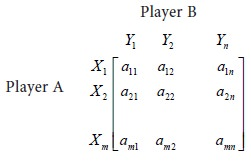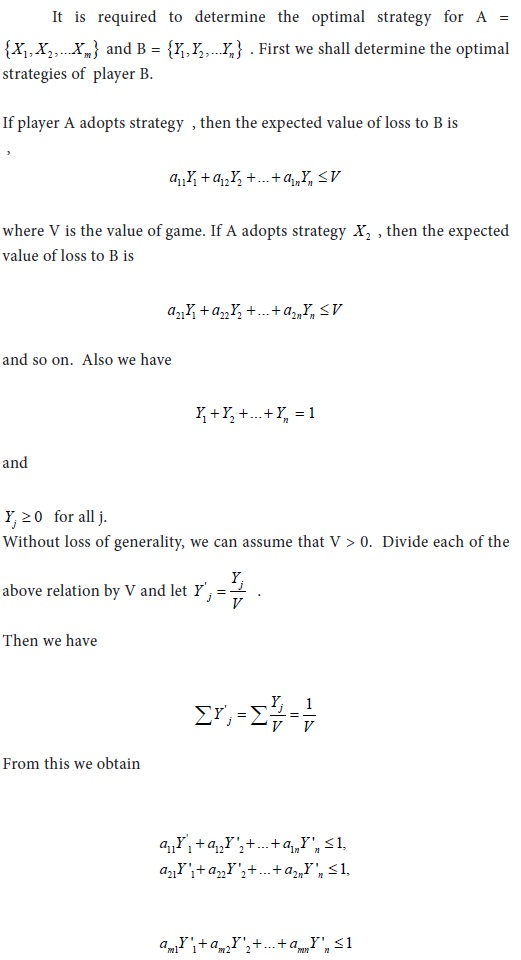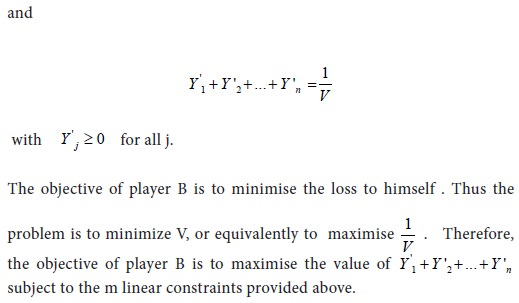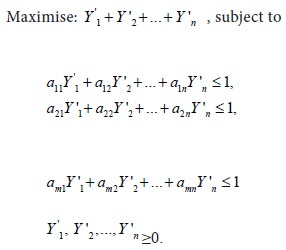Home | ARTS | Operations Management | Linear programming technique - Linear Programming Approach To Game Theory

# Linear programming technique - Linear Programming Approach To Game Theory

Posted On :  25.06.2018 01:22 am

When there is neither saddle point nor dominance in a problem of game theory and the payoff matrix is of order 3x3 or higher, the probability and graphical methods cannot be employed. In such a case, linear programming approach may be followed to solve the game.

Linear programming technique

A general approach to solve a game by linear programming technique is presented below. Consider the following game:## Statement of the problemWe can use simplex method to solve the above problem. For this purpose, we have to introduce non-negative slack variables s1 , s2 ,..., sm to each of the inequalities. So the problem can be restated as follows: Restatement of the problem:In a similar manner we can determine the optimal strategy for player A.

Application

We illustrate the method for a 2X2 zero sum game.

Tags : Operations Management - Game Theory, Goal Programming & Queuing Theory
Last 30 days 730 views